Courses

# Frequency Domain Analysis - 2 Notes | EduRev

## Electrical Engineering (EE) : Frequency Domain Analysis - 2 Notes | EduRev

The document Frequency Domain Analysis - 2 Notes | EduRev is a part of Electrical Engineering (EE) category.
All you need of Electrical Engineering (EE) at this link: Electrical Engineering (EE)

Stability Analysis

• If the Nyquist path in the s plane encircles Z zeros and P poles of [1 + G(s) H(s)] and does not pass through any poles or zeros of [1+ G(s) H(s)] as a representative point s moves in the clockwise direction along the Nyquist path, then the corresponding contour in the G(s) H(s) plane encircles the (–1 + j0) point N = Z – P times in the clockwise direction. (Negative values of N imply counterclockwise encirclements).
• In examining the stability of linear control system using the Nyquist stability criterion, we see that three possibilities  can occur.

1. There is no encirclement of the (– + j0) point. This implies that the system is stable if there are no poles of G(s)H(s) in the righthalf s-plane; otherwise, the system is unstable.

2. There is a counterclockwise encirclement or  encirclements of the –1 +j0 point. In this case the system is stable if the number of counterclockwise encirclements is the same as the number of poles of G(s)H(s) in the right-half a plane; otherwise, the system is unstable.

3. There is a clockwise encirclement or encirclements of the –1 +j0 point. In this case the system is unstable.

NOTE:
Encirclement: Used in Nyquist stability criteria. It is for closed path around (–1 + j0)

Enclosurement: Used in polar plot. A point in GH plane is said to be enclosed by the polar plot if it lies on the right hand side of plot when moving along the plot in the increasing direction of frequency from 0 to ∞ .

PHASE MARGIN & GAIN MARGIN

• Figure shows the polar plots of G(jω) for three different values of the open-loop gain K. For a large value of the gain K, the system is unstable. As the gain is decreased to a certain value, the G(jω) locus  passes through the – 1 –j0 point.
• This means that with this gain value the system is on the verge of instability, and the system is on the verge of instability, and the system will exhibit sustained oscillations. For a small value of the gain K, the system is stable. In general, the closer the G(jω) locus comes to encircling the –1 + j0 point, the more oscillatory is the systemresponse. The closeness of the G(jω) locus to the –1 + j0 point can be used as a measure of the margin of stability.
• This does not apply, however, to conditionally stable systems.
• It is  common practice to represent the closeness in terms of phase margin and gain margin.

Phase Margin

• The phase margin is that amount of additional phase lag at the gain crossover frequency required to bring the system to the verge of instability.
• The gain crossover frequency is the frequency at which |G( jw)| , the magnitude of the  openloop transfer function is unity.
• The phase margin g is 180º plus the phase angle f of the open-loop transfer function at the gain crossover frequency, or g = 180º + f.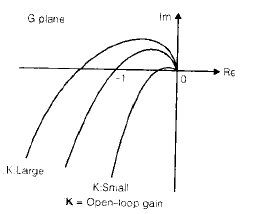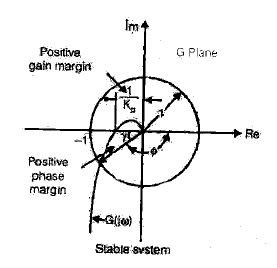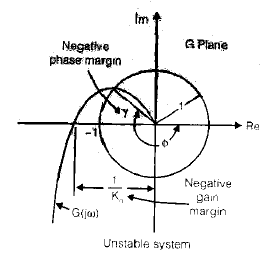• In the above figure of polar plot, a line may be drawn from the origin to the point at which the unit circle crosses the G(jω) locus.
• The angle from the negative real axis to his line is the phase margin. The phase margin is positive for g > 0 and negative for g < 0.  For minimum phase system to be stable, the phase margin must be positive.
• In the logarithmic plots, the critical point in the complex plane corresponds to the 0 dB and – 180º lines.

Gain Margin

• The gain margin is the reciprocal of the magnitude |G(jω)| at the frequency where the phase angle is – 180º.
• Defining the phase crossover frequency ω1 to be the frequency at which the phase angle of the open -loop transfer function equals –180º gives the gain margin Kg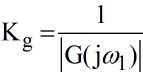in terms of decibels,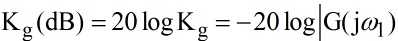• The gain margin expressed in decibels is positive if Kg is greater than unity and negative if Kg is smaller than unity.
• A positive gain margin (in decibels) means that the system is stable, and negative gain margin (in decibels) means that the system is unstable.  The gain margin and phase margins for different plots has been shown in figure (a), (b) and (c).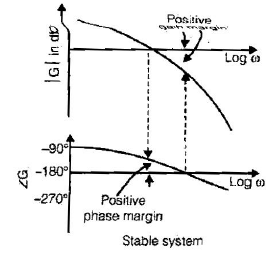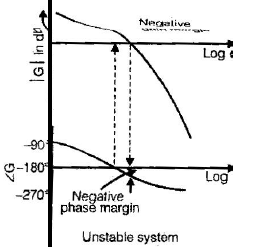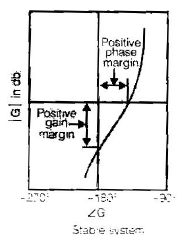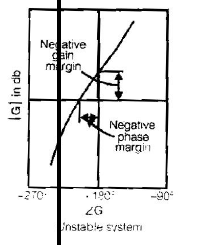(phase and gain margins of stable and unstable systems)

(a) Bode diagrams; (b) polar Plots; (c) log-magnitude versus phase plots.

• For a stable minimum phase system (defined in last section of this chapter), the  gain margin indicates how much the gain can be increased before the system becomes unstable.
• For an unstable system, the gain margin is indicative of how much the gain must be decreased to make the system stable.
• The gain margin of a first or second-order system is infinite since the polar plots for such systems do not cross the negative real axis. Thus, theoretically, first or second-order systems cannot be unstable.

NOTE:

• However, that so-called first or secondorder systems are only approximations in the sense thtat small time lags are neglected in deriving the system equations and are thus not truly first or second-order systems.
• If these small lags are accounted for, the so-called first or second order systems may become unstable.It is important to point out that for a nonminimum phase system the stability condition will not be satisfied unless the G(jω) plot encircles the (–1 + j0) point. Hence, a stable non-minimum phase system will have negative phase and gain margin.
• It is also imp ortant to point out that conditionally  stable  system will have two or more phase crossover frequencies, and some higher-order systems with complicated numerator dynamics may also have two or more gain crossover frequencies, as shown in Figure.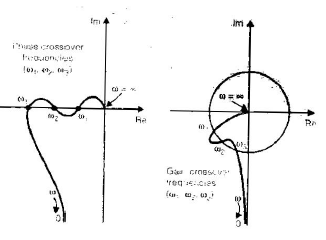• For stable systems having two or more gain crossover frequencies, the phase margin is measured at the highest gain crossover frequency.

A Few Comments on phase and Gain Margins

• The phase and gain margins of a control system are a measure of the closeness of the polar plot to the (–1 + j0) point. Therefore, these margins may be used as design criteria.
• It should be noted that either the gain margin alone or the phase margin alone does not give a sufficient indication of the relative stability. Both should be given in the determination of relative stability.
• For a minimum phase system, both the phase and gain margins must be positive for the system to be stable. Negative margins indicate instability.
• Proper phase and gain margins ensure us against variations in the system components and are specified for definite values of frequency. The two values bound the behavior of the closed loop system near the resonant frequency.
• For satisfactory performance, the phase margin should be between 30º and 60º, and the gain margin should be greater than 6 dB. with these values, a minimum phase system has guaranteed stability, even if the open-loop gain and time constants of the components very to a certain extent. Although the phase and gain margins given only rough estimates of the effective damping ratio of the closed-loop system, they do offer a convenient means for designing control systems or adjusting the gain constant of systems.

CLOSED LOOP FREQUENCY RESPONSE

• Let us define the magnitude of the closed-loop frequency response as M and the phase angle as α , or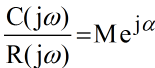• In the following, we shall find the constant magnitude loci and constant phase-angle loci. such loci are convenient in determining the closed-loop frequency response from the polar plot or Nyquist plot.

Constant magnitude Loci (M-circles) 

• to obtain the constant magnitude loci, let us first note that G(jω) is a complex quantity and can be written as follows: G(jω) = X + jY  where X and Y are real quantities. Then M is given by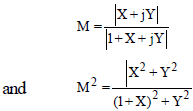Hence, X2 (1–M2)–2M2 X–M2 + (1 – M2)Y2 =0

• If M = 1, then from above equation (8.10) we obtain X =–1/2. This is the equations of a straight line parallel to the Y-axis and passing through the point (–1/2, 0).
• If M ≠ 1, equation can be written as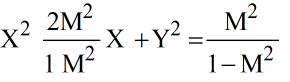if the term [M2/(1–M2)]2 is added to both sides of this last equation, we obtain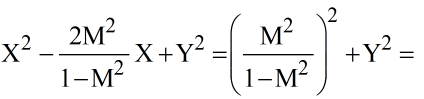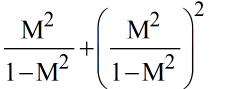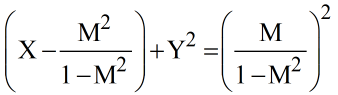• Equation (8.11) is the equation of a circle with centre at X = +M2/(M2–1), Y=0 and with radius |M / (M2 - 1)| .
• The constant M loci on the G(s) plane are thus a family of circles. The centre and radius of the circle for a given value of M can be easily calculated
• For M>1, the centers of the M circles lie to the left of the (–1 +j0) point. Similarly, as M becomes smaller compared with 1, the M circle becomes smaller and converges to the origin. For 0< M <1, the centers of the M circles lie to the right of the origin.
• M = 1 corresponds to the locus of points equidistant from the origin and from the (-1 + j0) point. As stated earlier, it is a straight line passing through the point (-1/2, 0) and parallel to the imaginary axis.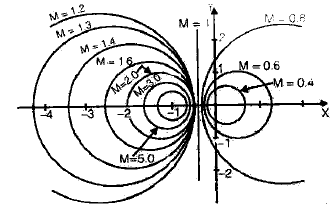• The M circles are symmetrical with respect to the straight line corresponding to M =1 and with respect to the real axis.

Constant Phase-angle Loci (N-circles)

• We shall obtain the phase angle α in terms of X and Y. Since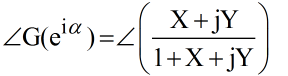the phase angle α is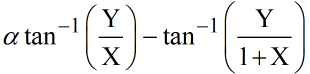if we define, tanα = N then,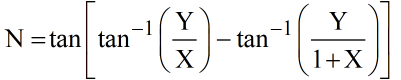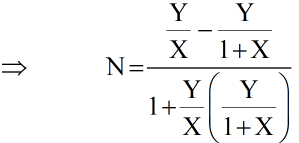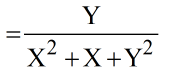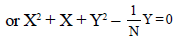The addition of (1/4) + 1/(2N)2 to both sides of this last equation yields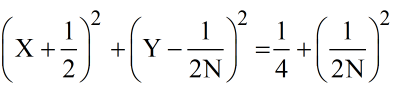This is an equation of a circle with centre at X = –1/2, Y = 1/(2N) and with radius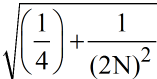Since equation is satisfied when X=Y=0 and X=–1, Y = 0 regardless of the value of N, each circle passes through the origin and the (–1 + j0) point. The constant N loci can be drawn easily once the value of N is given. A family of constant N circles are shown in figure below with a as a parameter.

It should be noted that the constant N locus for a given value of a is actually not the entire circle but only an arc. In other words, the α = 30º and α = 150º arcs are parts of the same circle. This is so because the tangent of an angle remains the same if ± 180º (or multiples of it) is added to the angle.

The use of the M and N circles enables us to find the entire closed-loop frequency response from the open-loop frequency response G(jω) without calculation the magnitude and phase of the closed-loop transfer function at each frequency. The intersections of the G(jω) locus and the M circles and N circles given the values of M and N at frequency points on the G(jω) locus. The N circles are multivalued in the sense that the circl e for α = α1 and that for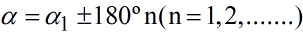are the same. In using the N circles for the determination of the phase angle of closed-loop systems, we must interpret the proper value of a. to avoid any error, start at zero frequency, which corresponds  to α = 0º, and proceed to higher frequencies. The phase-angle curve must be continuous.

• Graphically, the intersection of the G(jω) locus and M circles give the values of M at the frequencies denoted on the G(jω) locus. Thus, the constant M circle with the smallest radius that is tangent to the G(jω) locus gives the value of the resonant peak magnitude Mr. If it is desired to keep the resonant peak value less than  a certain value, then the system should not enclose the critical point (–1 + j0 point) and at the same time there should be no intersections with the particular M circle and the G(jω) locus.
• Figure (a) shows the G(jω) locus superimposed on a family of M circles. Figure (b) shows the G(jω) locus superimposed on family of N circles. From these plots, it is possible to obtain the closed loop frequency response by inspection. it is seen that the M = 1.1 circle intersects the G(jω) locus at frequency point ω = ω1. This means that at this frequency the magnitude of the closedloop transfer function is 1.1.
• In figure (a), the M = 2 circle is just tangent to the G(jω) locus. Thus, there is only one point on the G(jω) locus for which |C(jω) / R (jω)| is equal to 2. Figure (c) in the next page shows the closed loop frequency- response curve for the system. The upper curve is the M versus frequency w curve and the lower curve is the phase angle a versus frequency ω curve.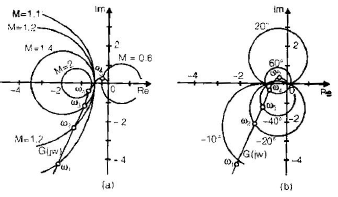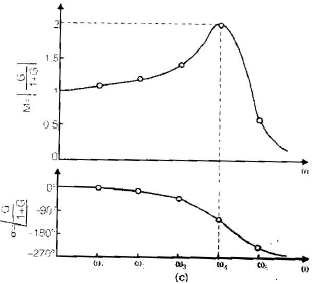• The resonant peak value is the value of M corresponding to the M circle of smallest radius that is tangent to the G(jω) locus. Thus, in the Nyquist diagram, the resonant peak value Mr and the resonant frequencywr can be found from the M-circle tangency to the G(jω) locus. (In the present example , Mr = 2 and ωr  = ω4).

General Procedure for Plotting Bode-Diagrams

• First rewrite the sinusoidal transfer function G(jω) H(jω) as a product of basic factors discussed above.
• Then identify the corner frequencies associated with these basic factors.
• Finally, draw the asymptotic log-magnitude curves with proper slopes between the corner frequencies. The exact curve, which lies close to the asymptotic curve, can be obtained by adding proper corrections.
• The phase-angle curve of G(jω) H(jω) can be drawn by adding the phase- angle curves of individual factors.

NOTE: The use of Bode diagrams employing asymptotic approximations requires much less time than other methods that may be used for computing the frequency response of a transfer function. The ease of plotting the frequencyresponse curves for a given transfer function and the ease of modification of the frequencyresponse curve as compensation is added are the main reasons why Bode diagrams are very frequently used in practice.

Offer running on EduRev: Apply code STAYHOME200 to get INR 200 off on our premium plan EduRev Infinity!

,

,

,

,

,

,

,

,

,

,

,

,

,

,

,

,

,

,

,

,

,

;